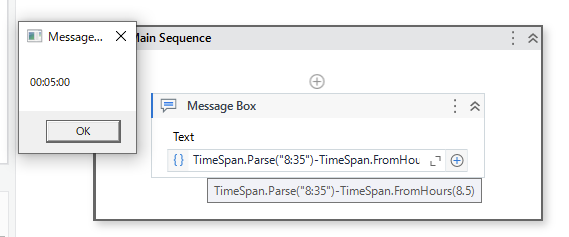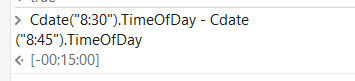# How to subtract 8.5 and 9:45?

Hi all,
I have table one column contains data like 8, 8.5 etc and second column contains 09:45, 8:35 etc. How to subtract 9:45 and 8 , 8.5 ad 8:35.
Can anyone solution.
Thank you.

@Vanitha_VS

May I know manually how you would subtract…like what is 8.5 and what is 8:35

cheers

Hi @Vanitha_VS ,

Use For each row activity to loop each row

Inside that use assign:

row(“Column3”) = CDbl(row(“Column2”)) - CDbl(row(“Column1”))

Actually 8.5 is 8:30(8 hr 30 min) and 8:35 is 8 hr 35 min

Hi,``````TimeSpan.Parse("8:35")-TimeSpan.FromHours(8.5)
``````

Regards,

2 Likes

@Vanitha_VS

Then use like this

But is 8:30 stored as 8:30 in the backend of excel…try reading excel usign workbook range and check then we can use this directly…else we need conversion again

`Cdate("8:30").TimeOfDay - Cdate("8:45").TimeOfDay`Else with Parsing can be used s suggested above

Hope this helps

cheers

This might help.

field1 = CDbl(row(“Column1”))
field2 = CDbl(row(“Column2”))

DateDiff(DateInterval.Minute,Cdate(Math.Floor(field1).tostring+“:”+((field1-Math.Floor(field1))*60).ToString),Cdate(Math.Floor(field2).tostring+“:”+((field2-Math.Floor(field2))*60).ToString))

Example:

DateDiff(DateInterval.Minute,Cdate(Math.Floor(8.5).tostring+“:”+((8.5-Math.Floor(8.5))*60).ToString),Cdate(Math.Floor(9.5).tostring+“:”+((9.5-Math.Floor(9.5))*60).ToString))

This gives output in minutes, if you want in hours make it DateInterval.Hour and for seconds DateInterval.Second,

Thank You. It is working fine.

1 Like

You can use the DateTime.Parse method to convert the time values to DateTime objects and then use the Subtract method.

``````DateTime time1 = DateTime.Parse("8:30");
DateTime time2 = DateTime.Parse("9:45");

TimeSpan difference = time2.Subtract(time1);
``````

This will give you a TimeSpan object representing the difference between the two times. You can then use the TotalMinutes property of the TimeSpan object to get the difference in minutes